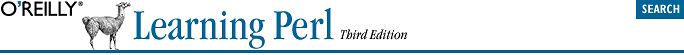home | O'Reilly's CD bookshelfs | FreeBSD | Linux | Cisco | Cisco Exam## 4.12. Exercises

See Section A.3, "Answers to Chapter 4 Exercises" for answers to the following exercises:

1.  Write a subroutine, called &total, which returns the total of a list of numbers. Hint: the subroutine should not perform any I/O; it should simply process its parameters and return a value to its caller. Try it out in this sample program, which merely exercises the subroutine to see that it works. The first group of numbers should add up to 25.

```my @fred = qw{ 1 3 5 7 9 };
my \$fred_total = &total(@fred);
print "The total of \@fred is \$fred_total.\n";
print "Enter some numbers on separate lines: ";
my \$user_total = &total(<STDIN>);
print "The total of those numbers is \$user_total.\n";```
2.  Using the subroutine from the previous problem, make a program to calculate the sum of the numbers from 1 to 1000.